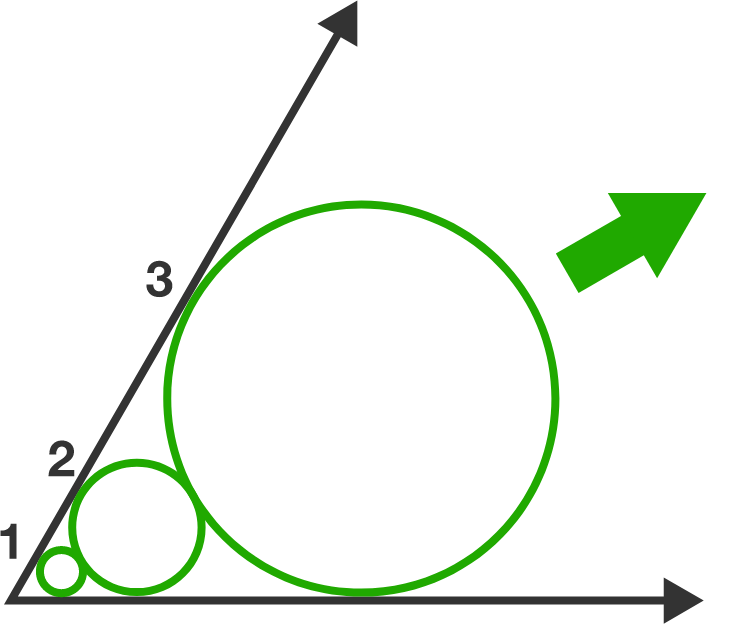# 2018 circles

Geometry Level 1The two rays in the diagram make an angle of $60^\circ.$ The first circle is tangent to both rays and has radius 1. Each successive circle is tangent to the two rays and externally tangent to the previous circle.

The radius of the 2018th is $a^b$, where $a$ and $b$ are positive integers with $b$ maximized.

What is $a+b$?

×